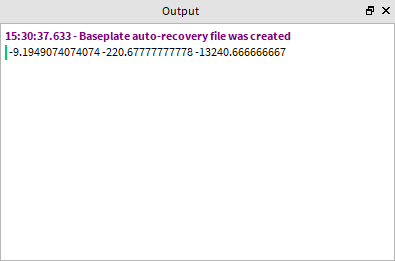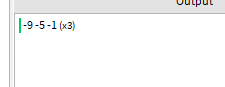# Countdown script can't get the day from "Nil"

I have a live event script:

``````local t = {
year = 2020,
month = 5,
day = 1,
hour = 15,
min = 50,
sec = 0}

local UnixTime = os.time(t)

while true do
wait(1)
local statistics = os.date("!*t", UnixTime - os.time())
print(statistics.year ..":".. statistics.month ..":".. statistics.day ..":".. statistics.hour ..":".. statistics.min ..":".. statistics.sec)
end
``````

It gets the unix time from a table and creates a formula to get the time left to be the same time as the table, in summary, a countdown. However, the issue is that an error is printing:
ServerScriptService.Script:15: attempt to index nil with ‘year’

The error is in the print, where it tries to get the year. I don’t know why this is happening, this is a script i got months ago, i don’t know if something is broken since before. Thanks for reading.

I’m pretty sure you need to use `os.difftime()` when subtracting time like that.
Print `UnixTime - os.time()` and see if that prints nil, and also try replacing the `-` with `os.difftime(UnixTime, os.time())`.

Also try running this function:

``````table.foreach(os.difftime(UnixTime, os.time()), print)
``````

`os.difftime(x, y)` does the operation `(x - y)`.

The issue looks like you’re subtracting incorrectly. You are trying to get the `os.date` of a negative number, which can not happen because `0` is EPOCH and nothing before will get counted.

If you want to get the amount of days until or from the dates in `t` you can do something like this:

``````local t = {
year = 2020,
month = 5,
day = 1,
hour = 15,
min = 50,
sec = 0
}

local UnixTime = os.time(t)

while true do
wait(1)
local diff = UnixTime - os.time()

local minutes = diff / 60
local hours = minutes / 60
local days = hours / 24

print(days, hours, minutes)
end
``````

Notice an output will be negative because `5/1/2020` was 9 days ago, or alternatively “-9” days from us.As @vtaetwrjxvdpgwjqkfzw said, i was substracting incorrectly, when i printed `UnixTime - os.time()`, it printed a negative number. The function you gave me didn’t work, it expected a table, however, i appreciate your time to help me.

The function i gave was for debugging, it wasn’t the actual solution btw

1 Like

When i changed the minute to 45, which was the moment where the current minute was 42, i tested the script and it wasn’t printingt printing the the time i expected: `-0 -3 -238`, i used math.ceil(), but i don’t think it would change the date. Is this happening because of the timezones?

I think what you actually wanted was the remaining times in terms of, if I have the day 5/1/2020 and today is 5/10/2020, I have 9 days, 4 hours, and 58 minutes ago. Is that correct?

Yeah, well, i have changed the date:

``````local t = {
year = 2020,
month = 5,
day = 10,
hour = 16,
min = 45,
sec = 0
}
``````

The moment when i tested this was at 16:42. And for any reason it was still printing negative.

Because you’re using universal time. Currently it is 20:53 at UTC-0

If you want to get the days, hours, and minutes between two times you can do something like this in the context that its the days, then hours remaining after the days are subtracted, then minutes remaining after the hours left have been subtracted:

``````local timeTable = {
year = 2020,
month = 5,
day = 1,
hour = 15,
min = 50,
sec = 0
}

local timeMeasurements = {
{"days", 60 * 60 * 24},
{"hours", 60 * 60},
{"minutes", 60}
}

local unixTime = os.time(timeTable)

local function timeUntil(timeStamp)
timeStamp = timeStamp - os.time()
local sign = math.sign(timeStamp)
timeStamp = math.abs(timeStamp)

local difference = {}

for i, measurement in pairs(timeMeasurements) do
local name = measurement
local value = measurement

local quantity = math.floor(timeStamp / value)
local remainder = timeStamp % value

difference[i] = sign * quantity

if (remainder == 0) then
break
end

timeStamp = remainder
end

return unpack(difference)
end

while true do
local days, hours, minutes = timeUntil(unixTime)

print(days, hours, minutes)
wait(1)
end
``````

5/1/2020 was 9 days ago, 5 hours ago, and 1 minute ago. (the minutes part is inaccurate but I’m not too sure how to fix that)1 Like